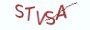Java基础教程

爬莲花山。

# 6.1.2 if, else if, else

```if (expression) {
;
} else if (expression){
;
} else {
;
}```

if语句定义条件控制的主干，expression表达式，表达式的值须为布尔类型。如果表达式的值为真值，那么就执行相应的操作。else if用来定义分支条件，即在if语句中的条件不成立的情况下，继续判断else if语句中的条件是否成立。else表示if语句和else if语句中的条件都不成立的情况下，才执行的操作。在条件控制的语法结构中，必须有一个if，可以有多个else if，但最多只有1个else。

```if (expression){
;
} else if (expression){
;
} else {
;
} else {
;
}```

```else if (expression){
;
} if (expression){
;
} else {
;
}  else if {
;
}```

```if (expression){
// 这是一个单独的条件控制结构
}
if (expression){
// 这是一个单独的条件控制结构
}```

# 6.1.3 条件表达式

```// 导入Random类来生成随机数
import java.util.Random;
public class HelloJava{
public  static void main(String[] args) {
// 构造Random对象以获取随机数
Random random = new Random();
// 通过Random对象的nextInt方法来获取指定范围内的正随机数
// 获取[0,100)区间内的正随机数
int x = random.nextInt(100);
int y = random.nextInt(100);
System.out.println("x:"+x+" y:"+y);
if (x > y) {
System.out.println("x > y");
} else {
System.out.println("x <= y");
}
}
}```

```// 导入Random类来生成随机数
import java.util.Random;
public class HelloJava{
public  static void main(String[] args) {
// 构造Random对象以获取随机数
Random random = new Random();
// 通过Random对象的nextInt方法来获取指定范围内的随机数
// 获取[0,100)之间的正随机数
int x = random.nextInt(100);
int y = random.nextInt(100);
// 如果x大于10，那么立即触发短路，不会再计算后面的表达式
if(x < 10 && x*y > 100) {
System.out.println("y一定大于10");
}else if ( x >= 10) {
System.out.println("y可能小于10");
}else {
System.out.println("y一定小于10");
}
}
}```

# 6.1.4 条件控制可以嵌套

```// 导入Random类来生成随机数
import java.util.Random;
public class HelloJava{
public  static void main(String[] args) {
// 构造Random对象以获取随机数
Random random = new Random();
// 通过Random对象的nextInt方法来获取指定范围内的随机数
// 获取[0,100)之间的正随机数
int x = random.nextInt(100);
int y = random.nextInt(100);

// 判断x与y的积是否大于10
if(x*y > 100) {
// 再嵌套一层if，来判断x是否小于10，如果x小于10，那么y一定大于10
if( x < 10){
System.out.println("y一定大于10");
} else {
System.out.println("y可能大于10");
}
}
}
}```

if嵌套的深度不宜过深，通常保持在三层以内即可。层数过多会导致代码的可读性变差，代码逻辑难以理解，难以维护。

# 6.1.5 Java的三元运算

expression ? expression1: expression2

```// 导入Random类来生成随机数
import java.util.Random;
public class HelloJava{
public  static void main(String[] args) {
// 构造Random对象以获取随机数
Random random = new Random();
// 通过Random对象的nextInt方法来获取指定范围内的随机数
// 获取[0,100)之间的正随机数
int x = random.nextInt(100);
int y = random.nextInt(100);
// 先假定x为最大值
int max = x;
if(x < y) {
// 如果x比y小，那么最大值一定是y
max = y;
}
System.out.println("最大值是:"+max);
}
}```

```// 导入Random类来生成随机数
import java.util.Random;
public class HelloJava{
public  static void main(String[] args) {
// 构造Random对象以获取随机数
Random random = new Random();
// 通过Random对象的nextInt方法来获取指定范围内的随机数
// 获取[0,100)之间的正随机数
int x = random.nextInt(100);
int y = random.nextInt(100);
// 如果x大于y,那么最大值就是x,否则是y
int max = x > y ? x:y;
System.out.println("最大值是:"+max);
}
}```

# 6.1.6 switch-case语句

switch -case也叫做开关语句，与if一样用作条件控制：根据不同的条件，执行不同的操作。switch-case的语法格式：

```switch( expression ){
case constExpression:
;
break;
case constExpression:
;
break;
default:
;
break;
}```

switch语句中的expression表达式必须输出整型，case语句中的constExpression表示常量表达式。Java在执行switch-case语句时，会按从上到下的顺序，将expression的值与case中的常量表达式进行匹配，直到匹配成功。

break语句用来停止继续往下进行匹配，即，如果不执行break,Java会继续判断下一个case中的常量表达式是否与expression的值匹配。

default用来定义所有case都不匹配的情况下才执行的操作。现在来写一个简单的程序，根据数字的奇偶性来做开关，一旦为偶数就输出芝麻开门，为奇数则输出芝麻关门，都不匹配就输出芝麻丢了：

```import java.util.Random;
public class HelloJava{
public  static void main(String[] args) {
Random random = new Random();
// 获取[0,10)之间的正随机数
int x = random.nextInt(10);
switch( x % 2){
// 先计算x%2表达式的值，然后与case中的常量进行匹配
case 0:
System.out.println("芝麻开门");
// 执行break会中止与下面case的匹配
break;
case 1:
System.out.println("芝麻关门");
break;
default:
System.out.println("芝麻丢了");
// 思考题:芝麻会丢吗？
break;
}
}
}```

6.1.7 课后习题

(1) 谈谈你对条件控制的理解。

(2) 条件控制的核心是什么？

(3) 假设有三个随机数:x,y,z，请编写条件控制语句来输出变量中的最大值和最小值。

(4) 假设有四个随机数:x,y,z,k,请编写条件控制语句来输出变量中的次大值和次小值。

(5) 将问题(4)和问题(5)中的程序通过三元运算符进行实现

(6) 将6.1.6节中的程序改成用if结构来实现。

# 6.1.8 高薪就业班

`(1) Python后端工程师高薪就业班，月薪10K-15K，免费领取课程大纲(2) Python爬虫工程师高薪就业班，年薪十五万，免费领取课程大纲(3) Java后端开发工程师高薪就业班，月薪10K-20K, 免费领取课程大纲(4) Python大数据工程师就业班，月薪12K-25K,免费领取课程大纲`## 注册账号